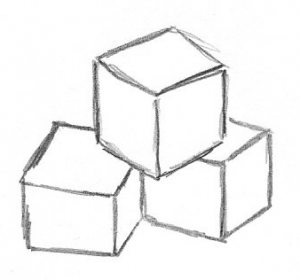# 42The number 42 is famous for its occurrence in The Hitchhiker’s Guide to the Galaxy. In 2032, Adele might come out with a new album with 42 as its title.  But today, the fame of the number 42 has to do with its representation as a sum of three cubes.

The problem is to try to represent each positive integer less than or equal to 100 as the sum of three cubes:  $x^3 + y^3 + z^3=n$, where $n$ is the given integer between 1 and 100 and $x$, $y$, and $z$ are the integers you need to find.  Some choices of $n$ are easy.  Some are known to be impossible, such as any $n$ that is equal to 4 or 5 mod 9.  Until recently, the answer was known for every $n$ except $n=33$ and $n=42$.  Then, in early 2019, Andrew Booker announced a representation of 33 as three cubes, using some good ideas and an enormous amount of computing time.  Hurray!  Booker was rightly celebrated for his result.  See, for example, the write-up in Quanta Magazine.  But the question remained for one more number: 42.

To try to crack 42, Booker teamed up with Andrew Sutherland.  Sutherland had a track record of breaking records using massively parallel computing.  Given the amount of time needed to solve 33, it seemed like parallel computing would be necessary (or at least a good idea) for 42.  They used Charity Engine, a global parallel computer that uses millions of idle personal PCs(*).  After some millions of hours of computer time, Booker and Sutherland came up with the answer:

$(-80538738812075974)^3 + (80435758145817515)^3 + (12602123297335631)^3 = 42$

Ta Dah!

The problem has a rich history, with lots of powerful ideas that produce integers with quite a few digits whose cubes add up to one- or two-digit numbers.  Reviews of a handful of papers on the subject are provided below.  There is also a nice video from Numberphile about the problem, which was posted to YouTube in November 2015, before Booker’s work on either 33 or 42, and a newer video posted just a few days ago, that discusses 42.

(*) The use of a global network of otherwise idle computers is also used in the hunt for Mersenne primes. The GIMPS project has been finding record large primes this way since 1996.

MR1850598
Elkies, Noam D.(1-HRV)
Rational points near curves and small nonzero $|x^3-y^2|$ via lattice reduction. (English summary) Algorithmic number theory (Leiden, 2000), 33–63,
Lecture Notes in Comput. Sci., 1838, Springer, Berlin, 2000.
11D75 (11G50 11H55 11J25)

The author gives an algorithm for effective calculation of all rational points of small height near a given plane curve $C$. Cases treated include the inequality $|x^3 + y^3 – z^3| < M \quad (0 < x \le y < z < N)$. Its solutions can be enumerated in heuristic time $\ll M\log^CN$ provided $M \gg N$, using $O(\log N)$ space. The algorithm is adapted to find all integer solutions of $0 < |x^3 – y^2| \ll x^{1/2}$ with $x < X$ in (rigorous) time $O(X^{1/2} \log^{O(1)}X)$. The strength of the algorithm, which involves linear approximation to the curve and lattice reduction, is illustrated by an example of integers $x,y$ with $x^{1/2}/|x^3 – y^2|$ greater than 46. The previous record was 4.87. The author discusses variations and generalizations. For example, fix an algebraic curve $C/\Bbb{Q}$ and a divisor $D$ on $C$ of degree $d > 0$. One can find the points of $C$ whose height relative to $D$ is at most $H$ in time $A(\epsilon)H^{(2/d)+\epsilon}$. Here $A(\epsilon)$ is effectively computable for each $\epsilon > 0$.

{For the collection containing this paper see MR1850596.}

Reviewed by R. C. Baker

MR1146835
Heath-Brown, D. R.(4-OXM)
The density of zeros of forms for which weak approximation fails.
Math. Comp. 59 (1992), no. 200, 613–623.
11G35 (11D25 11P55)

Let $f_k(x_1,x_2,x_3,x_4)=x^3_1+x^3_2+x^3_3-kx^3_4$. The author shows that if ${\bf x}$ is a primitive solution of $f_2({\bf x})=0$, then one of $x_1,x_2,x_3$ is divisible by 6. Thus $f_2$ has no rational zero close to both $(0,1,1,1)\in{\bf Q}^4_2$ and $(1,0,1,1)\in{\bf Q}^4_3$. Since these are zeros of $f_2$, the “weak approximation” principle fails for $f_2$. He obtains an analogous result for $f_3$.

Let $R_k(N)=\#\{{\bf x}\in{\bf Z}^4\colon\;\max_{i\leq 3}|x_i|\leq N$${\bf x}$ primitive, $f_k({\bf x})=0\}$. The author computes $R_k(1000)$ $(k=2,3)$ and observes some agreement with the hypothetical Hardy-Littlewood formula (1) $R_k(N)\sim {\frak S}_kN$. Subject to a conjecture on uniform convergence of certain products, he establishes that $\sum_{K<k\leq 2K}^*R_k(N)\sim N\sum^*_{K<k\leq 2K}{\frak S}_k$, where $\sum^*$ indicates omission of cubes. Perhaps, despite failure of the weak approximation principle, (1) is correct.

Reviewed by R. C. Baker

MR0000026
Davenport, H.
On Waring’s problem for cubes.
Acta Math. 71, (1939). 123–143.
10.0X

There are very few results concerning Waring’s problem which may be called best possible. One of these is the main theorem of this paper: If $E_s(N)$ denotes the number of positive integers not greater than $N$ which are not representable as a sum of $s$ positive integral cubes, then $E_4(N)/N\to 0$ as $N\to \infty$. A simple argument shows that the theorem is false if $s < 4$.

The new weapon used here has been explained in detail in several papers by the author [C. R. Acad. Sci. Paris 207, 1366 (1938); Proc. Roy. Soc. London 170, 293–299 (1939); Ann. of Math. 40, 533–536 (1939)]. The remainder of the proof is similar to that given by Landau [Vorlesungen über Zahlentheorie, Bd. 1, 235–303] for the third Hardy-Littlewood theorem [Satz 346] as adapted by Davenport and Heilbronn [Proc. London Math. Soc. (2) 43, 73–104 (1937)] to the problem of representations by two cubes and one square. Interesting parts of the proof are the use of Poisson’s summation formula in Lemma 7 and the handling of the Singular Series.

Reviewed by R. D. James